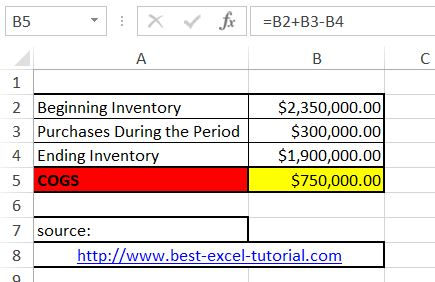#### How to calculate COGS?

In this Excel tutorial, you can learn how to calculate COGS in Excel.

## What is COGS?

Cost of goods sold (COGS) is the cost of the products or services that a business sells. In Excel, you can calculate COGS by subtracting the ending inventory from the cost of goods purchased.

Let’s build the cost of goods sold calculator in Excel. To calculate COGS in Excel, first you need some data. You need: Beginning Inventory, Purchases During the Period and Ending Inventory.

## How to calculate?

The formula to calculate COGS is as follows:

Cost of Goods Sold = Beginning Inventory + Purchases During the Period – Ending Inventory

Copy and paste this COGS formula in cell B5: =B2+B3-B4This formula will calculate the COGS for data you place in cells B2, B3 and B4 and is based on the cogs formula:

COGS= Inventory difference + Purchases

Note: You can extend this example by adding additional rows to calculate COGS for multiple periods or by using the COGS calculation in other formulas to analyze your business’s financial performance.

Excel can also be used to forecast future COGS based on sales projections and inventory levels. This helps in budgeting and planning for future financial needs.

You can create dynamic models in Excel that automatically adjust COGS calculations as sales and inventory data change.

Implement data validation and error-checking techniques in your Excel spreadsheets to prevent calculation errors and data inconsistencies.